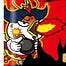William BrennanAug 19, 2022Liked by Dylan BlackIt seems unlikely that classical and medieval generals (or anyone at that time) had the mathematical knowledge to optimize this with the harmonic, though it's possible that it could have been worked out by combining many different generals' trial and error.Expand full commentI got a similar improvement over pairwise transfer using a different protocol.Transferring food between donkeys takes a fair amount of work, so probably has to be done every evening in the camp rather than whenever they've traveled L0/n. So let's suppose that transfers happen at regular intervals.I need a variable for the transfer rate. For simplicity, it will be N, defined as follows. If transfers happen every distance l, let N=L0/l. If transfers instead happen every time t, let N=f0/δt. The two-donkey protocol is then N=2.So at each transfer, the donkeys have eaten 1/N of the food they are carrying, so 1/N of the donkeys must be released so that the remaining (N-1)/N donkeys, now carrying (N-1)/N of the food they had at the last transfer, all now have full loads.(You are making the simplifying assumption that transfers can be done at any time. I am making the different simplifying assumption that we always have so many donkeys that, when we release 1/N of the remaining donkeys, rounding off to a whole number introduces negligible error.)So to travel a distance L0, the donkeys must make N transfers, and thus have ((N-1)/N)^N as many donkeys left at the end as when they started. Put another way, if you want to have D (full) donkeys after traveling a distance L, then you must start out with D(N/(N-1))^(NL/L0).So the key is that ratio B(N)=(N/(N-1))^N. It turns out that B(2)=4 is pretty bad. Observe:B(2)=4B(3)=3.375B(4)=3.16049382716049B(6)=2.985984B(8)=2.91028536804653B(12)=2.84094437661549B(16)=2.80840396557645B(30)=2.76501635584221B(60)=2.7412858509404B(100)=2.73199902642904. This is probably the practical limit in that it represents doing a transfer every night on wagons that can carry 100 days' worth of food, and is pretty close to the theoretical limit of e=2.71828.For L/L0=10, at the right end of your graphs, the harmonic donkey protocol needs to start out with over 10000 donkeys—most of the transfers look more like the B(12) or B(30) or B(100) case than the B(2) case. 4^10 = 1048576, 3^10 = 59049—even the six-donkey protocol is giving you an almost 18-fold improvement over the two-donkey protocol.So yes, I believe in your last graph. It's an exponential graph with base (4/e).Expand full commentWhat about grass on the side of the road? I think herd animals tend be good at "in-situ" resource collection. Also water probably weighs as much as food. Expand full commentThe Mongol range was far greater than any other military until the British Empire. Their solution? Soldiers drank the milk of the mares, which could produce milk for roughly 10 days on standard grass before they need a period of grazing. Mongols planned military campaigns around first securing foraging within 1-2 days of the target city, often splitting forces per available foraging. That would be an interesting equation, but heuristics seemed to work well enough. Expand full commentThere should be straight-forward extension to the polar explorer problem using dogs and/or horses, which are eaten when other supplies are exhausted. It's a little more complicated because the task of hauling sleds is transferred from the animals to the explorers as the animals are consumed. Unfortunately, the margin of my brainpower is too small to contain the derivation of this.Expand full commentAnother way to carry food energy is to store it in adipose tissue (fat). I wonder if this was ever used as an intentional strategy. It is a net loss to fatten up a soldier who dies though, so you would need to factor in loss rates in addition to the obvious question of fighting effectiveness with increased adiposity.Do donkeys get fat? Expand full commentCan't wait for the relativistic version!Expand full comment